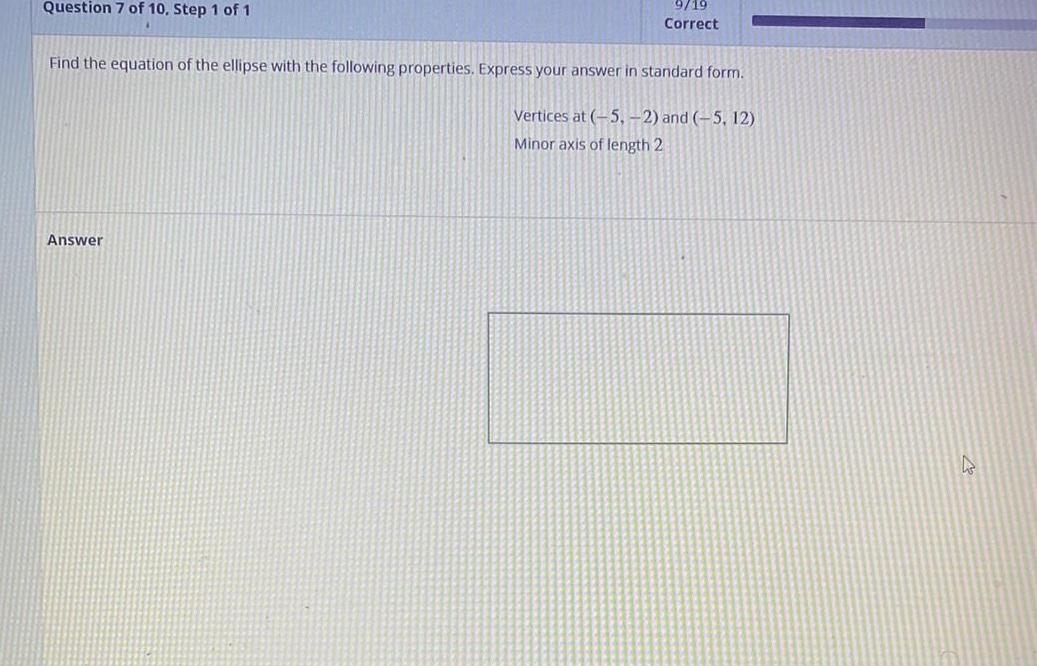Math
Ellipse
Find the equation of the ellipse with the following properties. Express your answer in standard form. Vertices at (-5, -2) and (-5, 12) Minor axis of length 2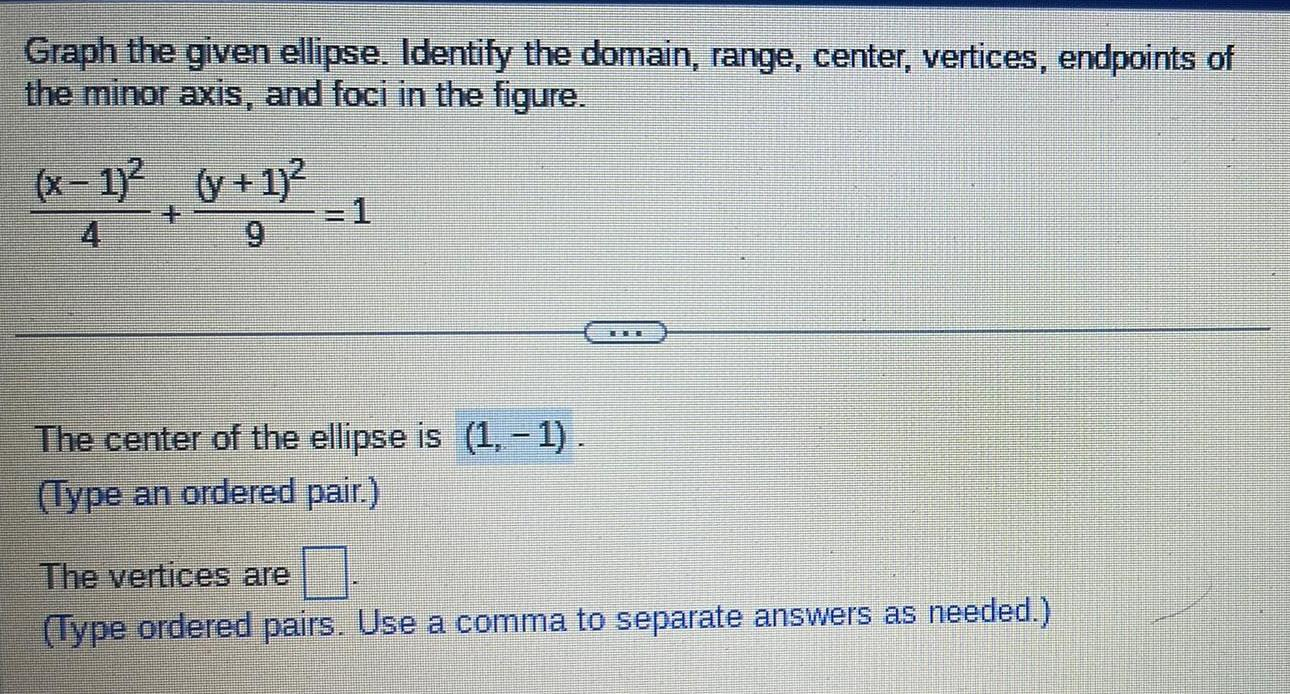Math
Ellipse
Graph the given ellipse. Identify the domain, range, center, vertices, endpoints of the minor axis, and foci in the figure. (x-1)²/4 + (y + 1)²/9 =1 The center of the ellipse is (1,-1). (Type an ordered pair.) The vertices are . (Type ordered pairs. Use a comma to separate answers as needed.)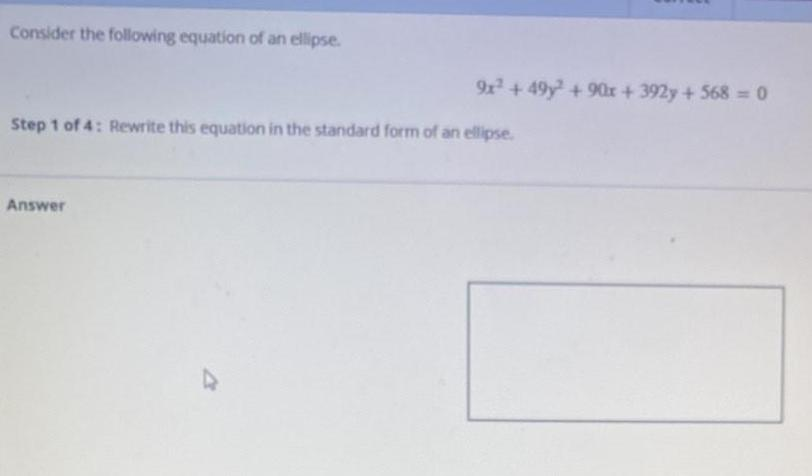Math
Ellipse
Consider the following equation of an ellipse. 9x² + 49y² +90x+ 392y + 568 = 0 Step 1 of 4: Rewrite this equation in the standard form of an ellipse. Answer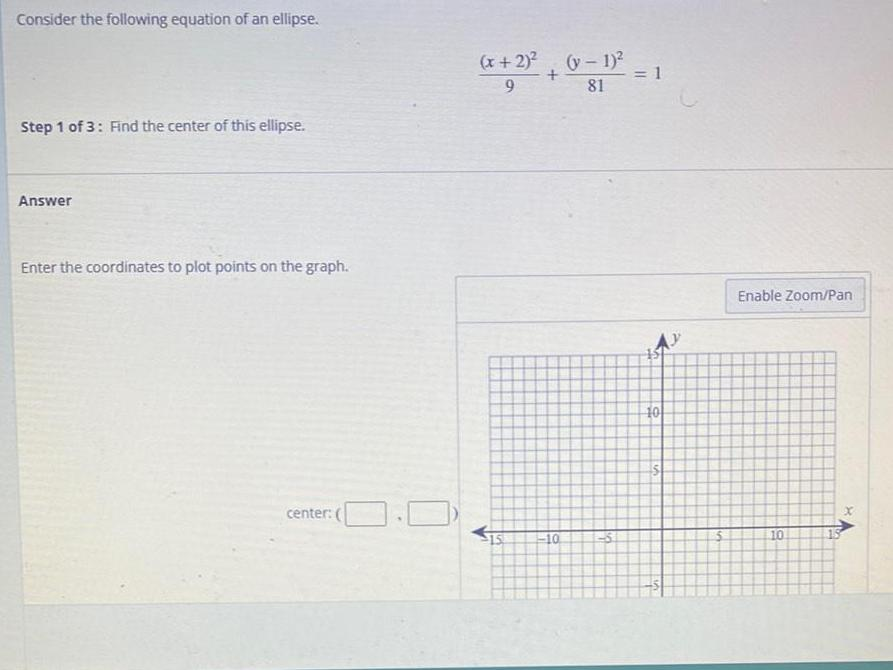Math
Ellipse
Consider the following equation of an ellipse. (x+2)^2 /9 + (y-1)^2 /81 =1 Find the center of this ellipse. Answer Enter the coordinates to plot points on the graph. center: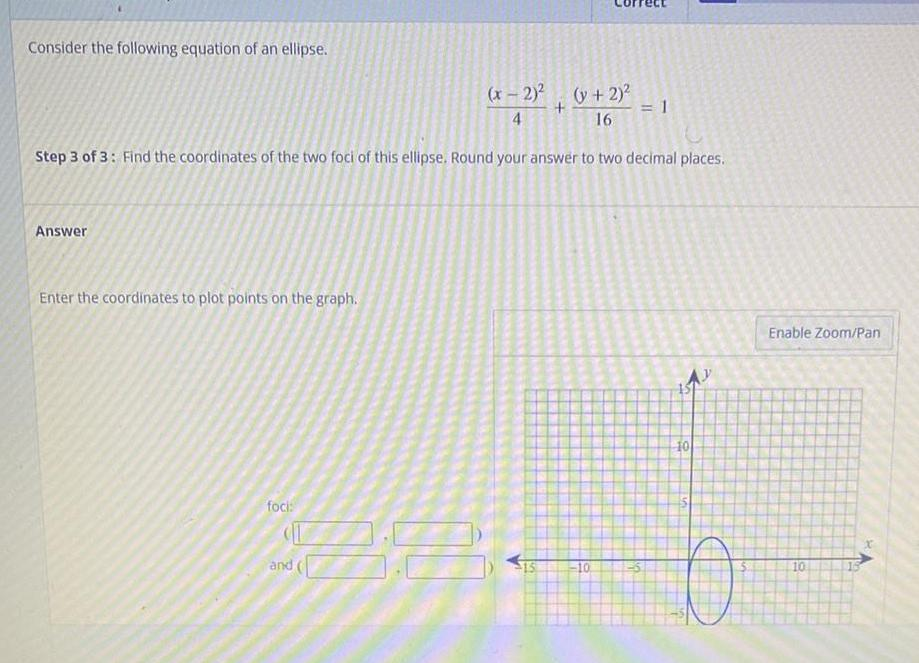Math
Ellipse
Consider the following equation of an ellipse. (x - 2)²/4+(y + 2)² /16 =1 Step 3 of 3: Find the coordinates of the two foci of this ellipse. Round your answer to two decimal places Enter the coordinates to plot points on the graph.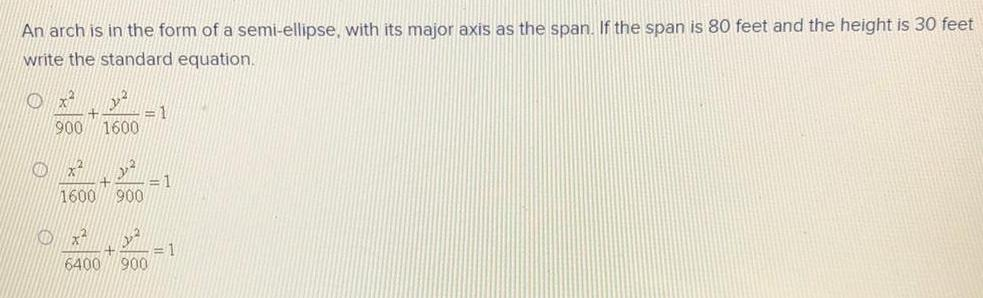Math
Ellipse
An arch is in the form of a semi-ellipse, with its major axis as the span. If the span is 80 feet and the height is 30 feet write the standard equation. x²/900+ y²/1600=1 x²/1600+ y²/900=1 x²/6400+ y²/900=1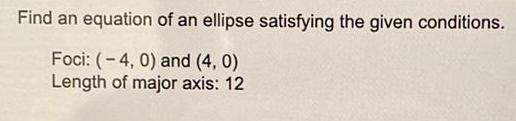Math
Ellipse
Find an equation of an ellipse satisfying the given conditions. Foci: (-4, 0) and (4, 0) Length of major axis: 12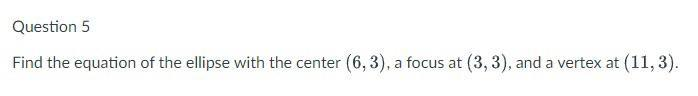Algebra
Ellipse
Find the equation of the ellipse with the center (6,3), a focus at (3,3), and a vertex at (11,3).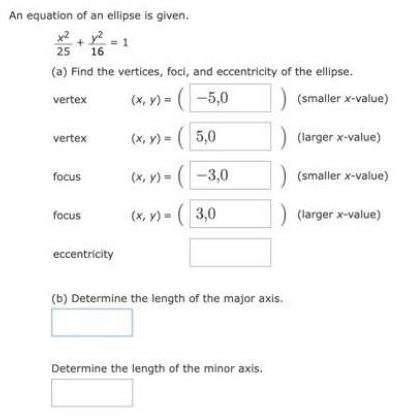Math
Ellipse
An equation of an ellipse is given. x2/25 + y²/16 = 1 25 16 (a) Find the vertices, foci, and eccentricity of the ellipse.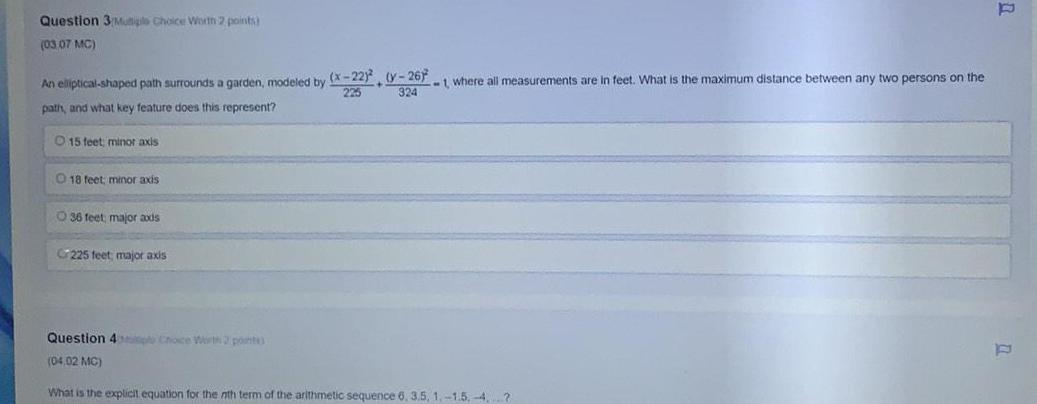Math
Ellipse
An elliptical-shaped path surrounds a garden, modeled by (x-22)/225+ (y-26)²/324 =1, where all measurements are in feet. What is the maximum distance between any two persons on the path, and what key feature does this represent? 15 feet; minor axis 18 feet; minor axis 36 feet major axis 225 feet; major axis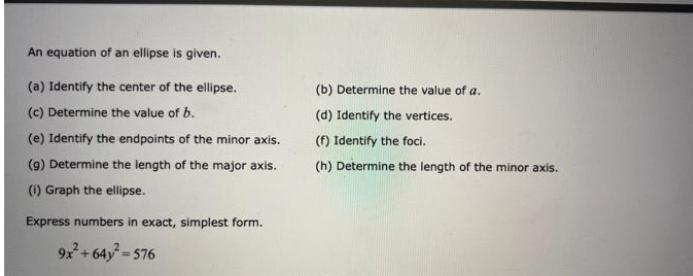Math
Ellipse
An equation of an ellipse is given. (a) Identify the center of the ellipse. (b) Determine the value of a. (c) Determine the value of b. (d) Identify the vertices. (e) Identify the endpoints of the minor axis. (f) Identify the foci. (g) Determine the length of the major axis. (h) Determine the length of the minor axis. (i) Graph the ellipse. Express numbers in exact, simplest form. 9x^2 + 64y^2 = 576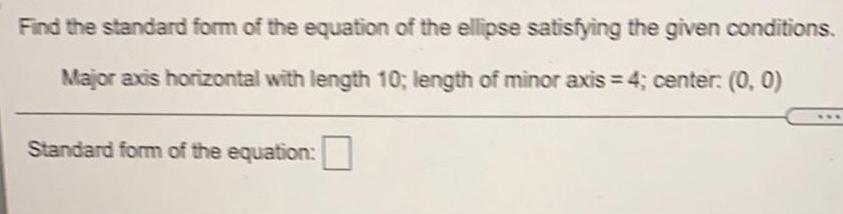Math
Ellipse
Find the standard form of the equation of the ellipse satisfying the given conditions. Major axis horizontal with length 10; length of minor axis = 4; center: (0, 0). Standard form of the equation: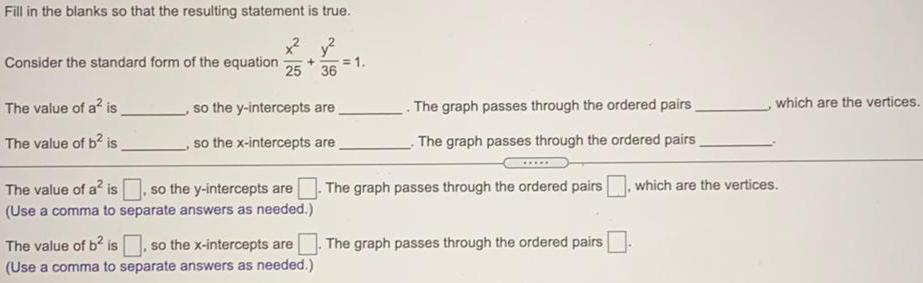Math
Ellipse
Consider the standard form of the equation x²/25 + y²/36 = 1 The value of a² is, so the y-intercepts are The graph passes through the ordered pairs which are the vertices. The value of b² is, so the x-intercepts are The graph passes through the ordered pairs The value of a² is, so the y-intercepts are The graph passes through the ordered pairs , which are the vertices. The value of b² is, so the x-intercepts are The graph passes through the ordered pairs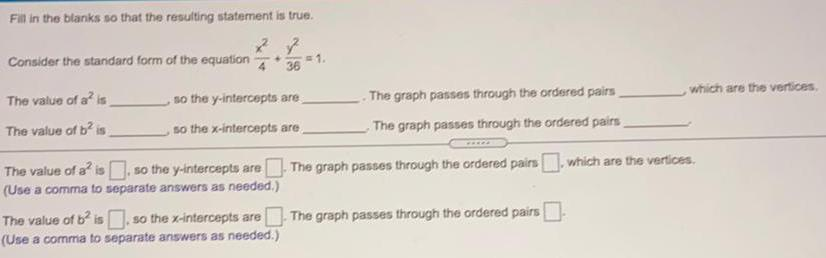Math
Ellipse
Fill in the blanks so that the resulting statement is true. Consider the standard form of the equation x2 4 + y2 36 = 1 The value of a² is so the y-intercepts are The graph passes through the ordered pairs, which are the vertices. The value of b² is so the x-intercepts are The graph passes through the ordered pairs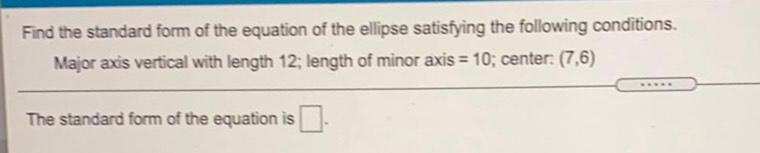Math
Ellipse
Find the standard form of the equation of the ellipse satisfying the following conditions. Major axis vertical with length 12; length of minor axis = 10; center: (7,6) The standard form of the equation is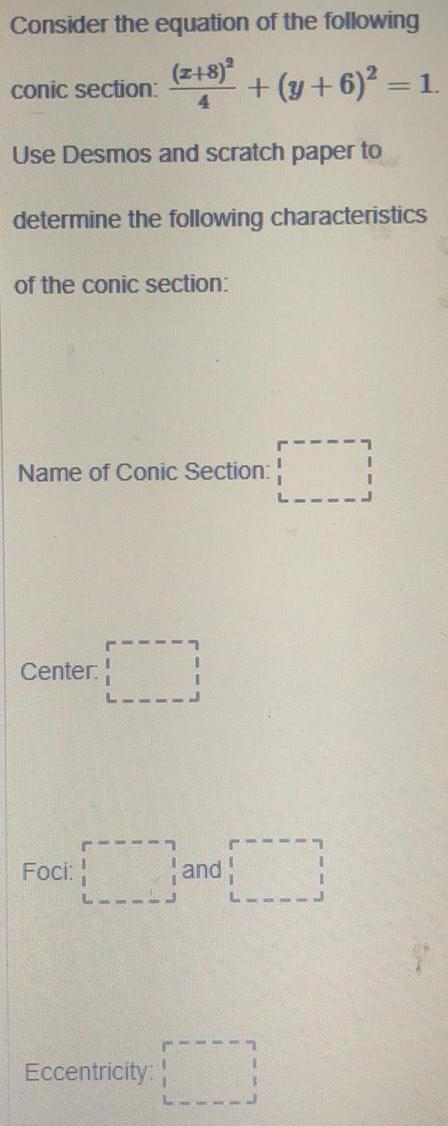Math
Ellipse
Consider the equation of the following (x+8)²/4 conic section: + (y + 6)² = 1. Use Desmos and scratch paper to determine the following characteristics of the conic section: Name of Conic Section: Center. Foci: Eccentricity: